# Announcements Coronavirus COVID19 Take care of yourself and

• Slides: 34Announcements Coronavirus – COVID-19 § Take care of yourself and others around you § Follow CMU and government guidelines § We’re “here” to help in any capacity that we can § Use tools like zoom to communicate with each other too! Zoom § Let us know if you have issues § Etiquette: Turn on video when talking or your turn in OH Feedback: See Piazza post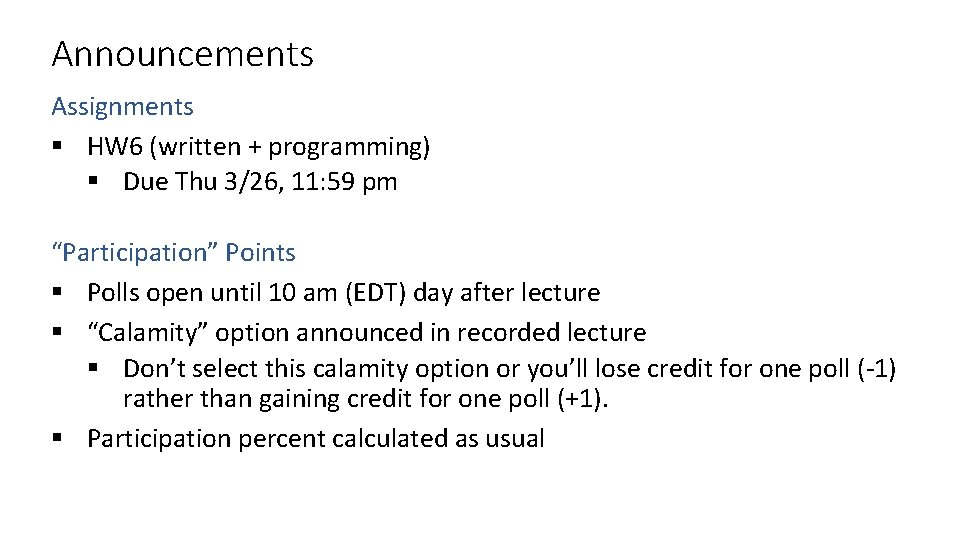Announcements Assignments § HW 6 (written + programming) § Due Thu 3/26, 11: 59 pm “Participation” Points § Polls open until 10 am (EDT) day after lecture § “Calamity” option announced in recorded lecture § Don’t select this calamity option or you’ll lose credit for one poll (-1) rather than gaining credit for one poll (+1). § Participation percent calculated as usualIntroduction to Machine Learning Cross-Validation Nonparametric Regression Instructor: Pat VirtueValidation Why do we need validation? § Choose hyperparameters § Choose technique § Help make any choices beyond our parameters But now, we have another choice to make! § How do we split training and validation? Trade-offs § More held-out data, better meaning behind validation numbers § More held-out data, less data to train on!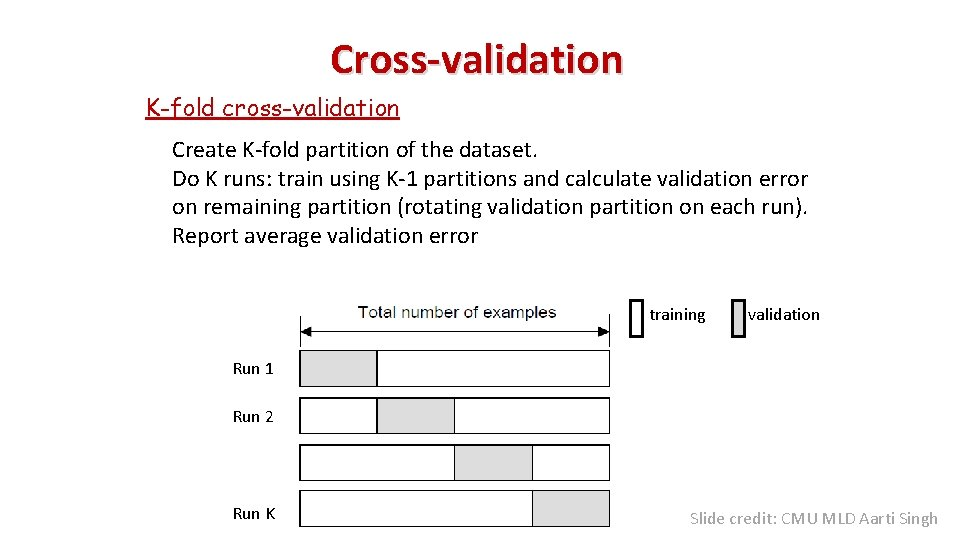Cross-validation K-fold cross-validation Create K-fold partition of the dataset. Do K runs: train using K-1 partitions and calculate validation error on remaining partition (rotating validation partition on each run). Report average validation error training validation Run 1 Run 2 Run K Slide credit: CMU MLD Aarti SinghCross-validation Leave-one-out (LOO) cross-validation Special case of K-fold with K=N partitions Equivalently, train on N-1 samples and validate on only one sample per run for N runs training validation Run 1 Run 2 Run K Slide credit: CMU MLD Aarti SinghCross-validation Random subsampling Randomly subsample a fixed fraction αN (0< α <1) of the dataset for validation. Compute validation error with remaining data as training data. Repeat K times Report average validation error training validation Run 1 Run 2 Run K Slide credit: CMU MLD Aarti Singh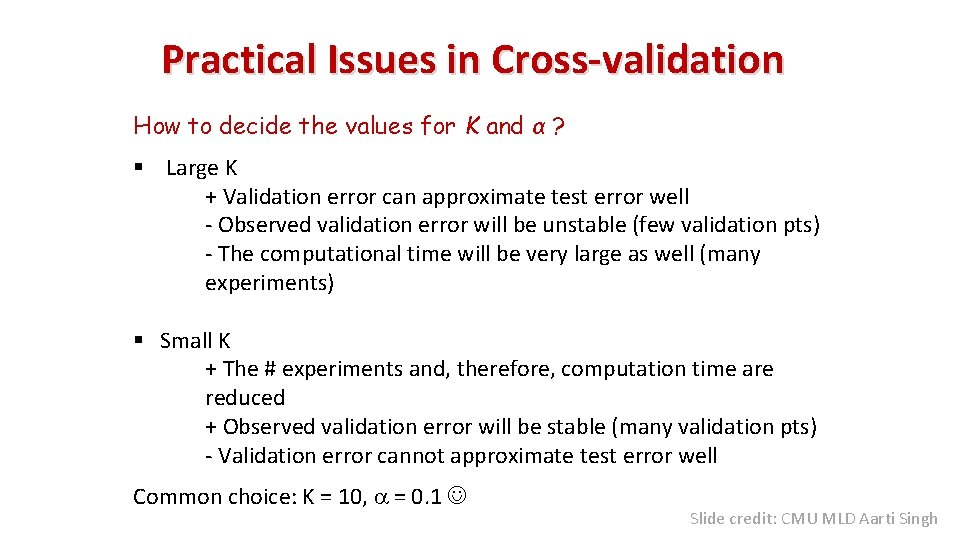Practical Issues in Cross-validation How to decide the values for K and α ? § Large K + Validation error can approximate test error well - Observed validation error will be unstable (few validation pts) - The computational time will be very large as well (many experiments) § Small K + The # experiments and, therefore, computation time are reduced + Observed validation error will be stable (many validation pts) - Validation error cannot approximate test error well Common choice: K = 10, a = 0. 1 Slide credit: CMU MLD Aarti SinghPiazza Poll 1 Say you are choosing amongst 10 values of lambda, and you want to do K=10 fold cross-validation. How many times do I have to train my model? A. 0 B. 1 C. 10 D. 20 E. 100 F. 1010Output y Nonparametric Regression Input x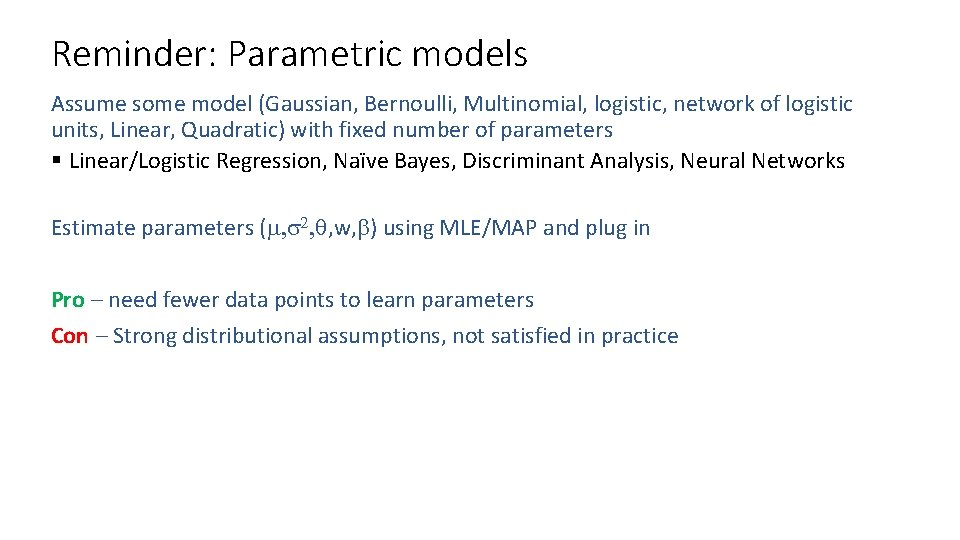Reminder: Parametric models Assume some model (Gaussian, Bernoulli, Multinomial, logistic, network of logistic units, Linear, Quadratic) with fixed number of parameters § Linear/Logistic Regression, Naïve Bayes, Discriminant Analysis, Neural Networks Estimate parameters (m, s 2, q, w, b) using MLE/MAP and plug in Pro – need fewer data points to learn parameters Con – Strong distributional assumptions, not satisfied in practice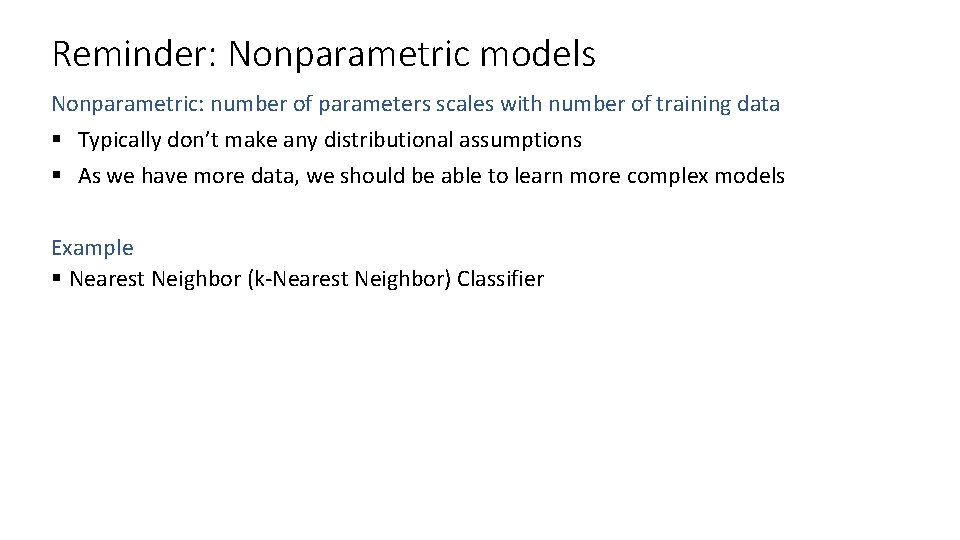Reminder: Nonparametric models Nonparametric: number of parameters scales with number of training data § Typically don’t make any distributional assumptions § As we have more data, we should be able to learn more complex models Example § Nearest Neighbor (k-Nearest Neighbor) Classifier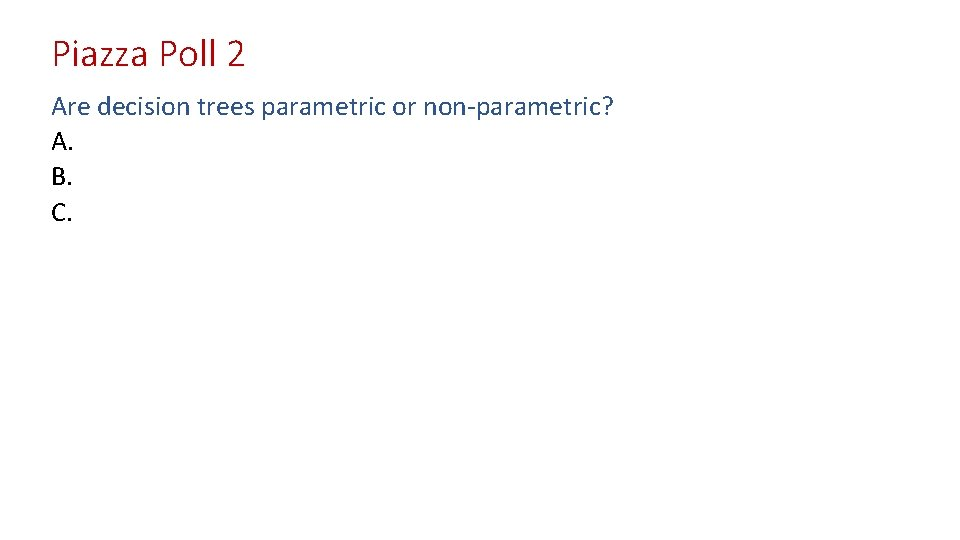Piazza Poll 2 Are decision trees parametric or non-parametric? A. B. C.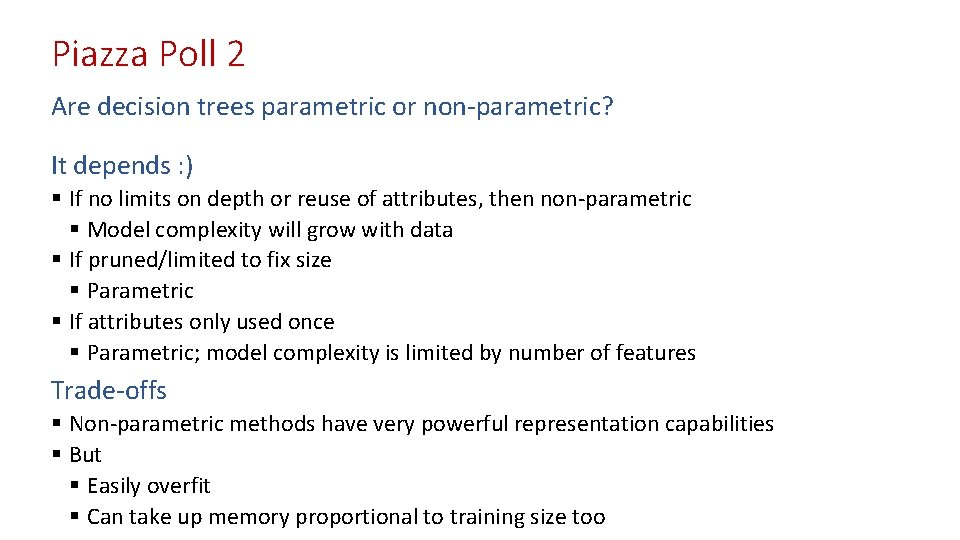Piazza Poll 2 Are decision trees parametric or non-parametric? It depends : ) § If no limits on depth or reuse of attributes, then non-parametric § Model complexity will grow with data § If pruned/limited to fix size § Parametric § If attributes only used once § Parametric; model complexity is limited by number of features Trade-offs § Non-parametric methods have very powerful representation capabilities § But § Easily overfit § Can take up memory proportional to training size tooNonparametric Regression Output y Decision Trees Input x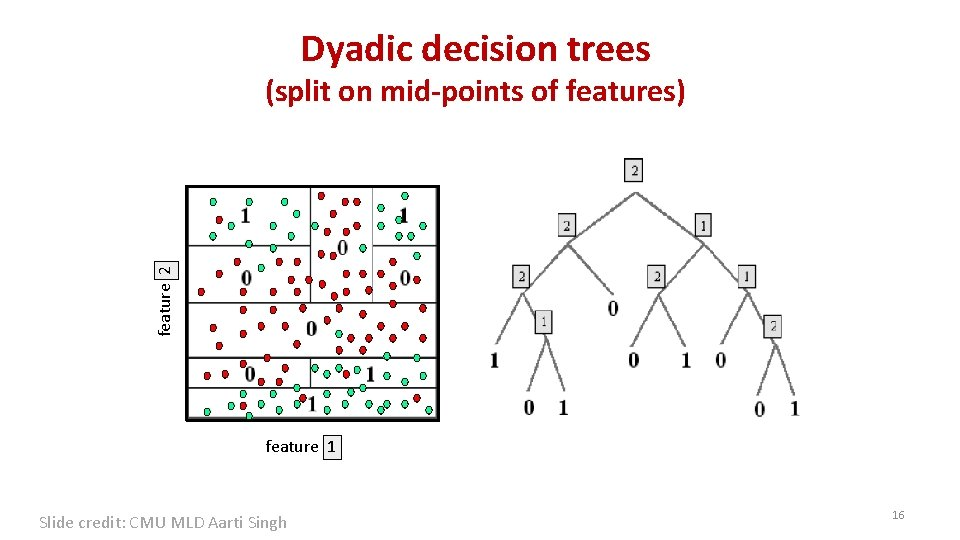Dyadic decision trees feature 2 (split on mid-points of features) feature 1 Slide credit: CMU MLD Aarti Singh 16How to assign label to each leaf Classification – Majority vote Regression – Constant/Linear/Poly fit 17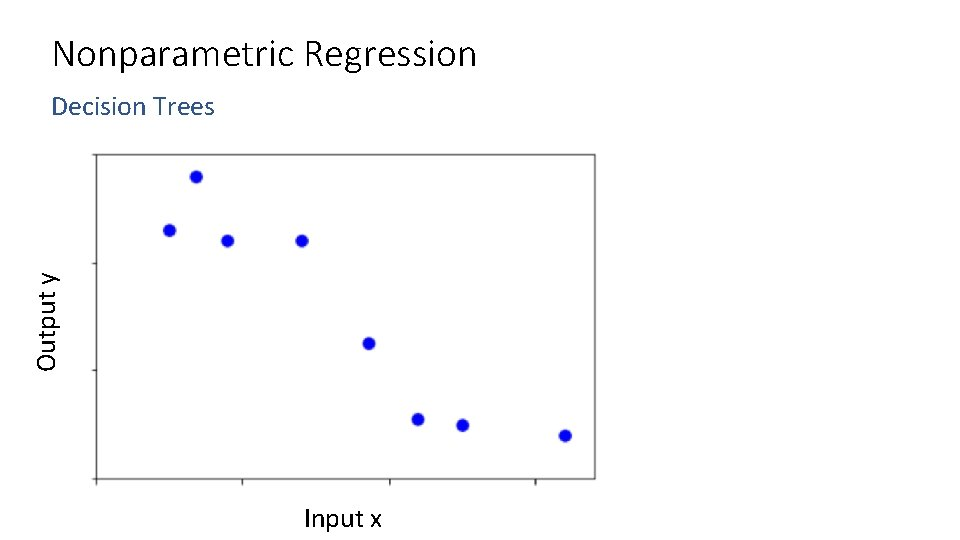Nonparametric Regression Output y Decision Trees Input x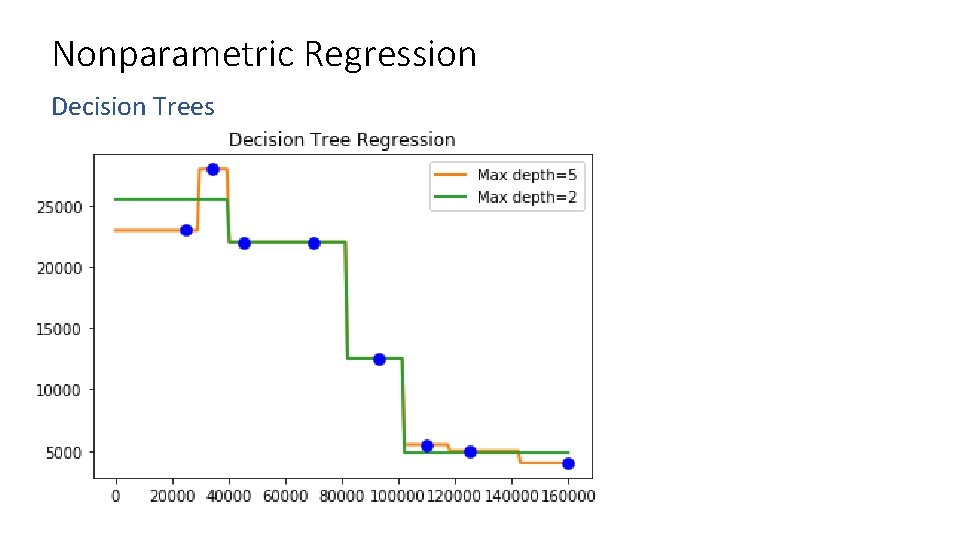Nonparametric Regression Decision Trees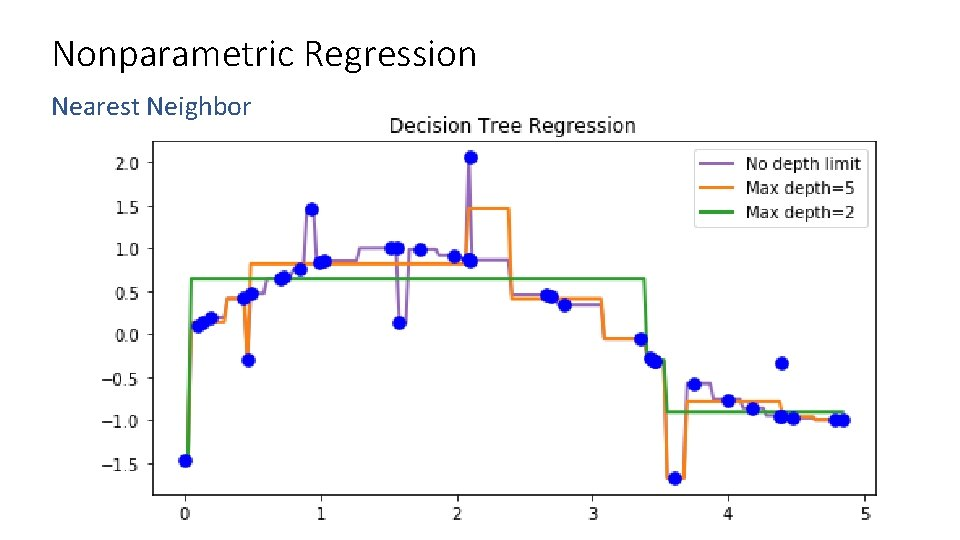Nonparametric Regression Nearest Neighbor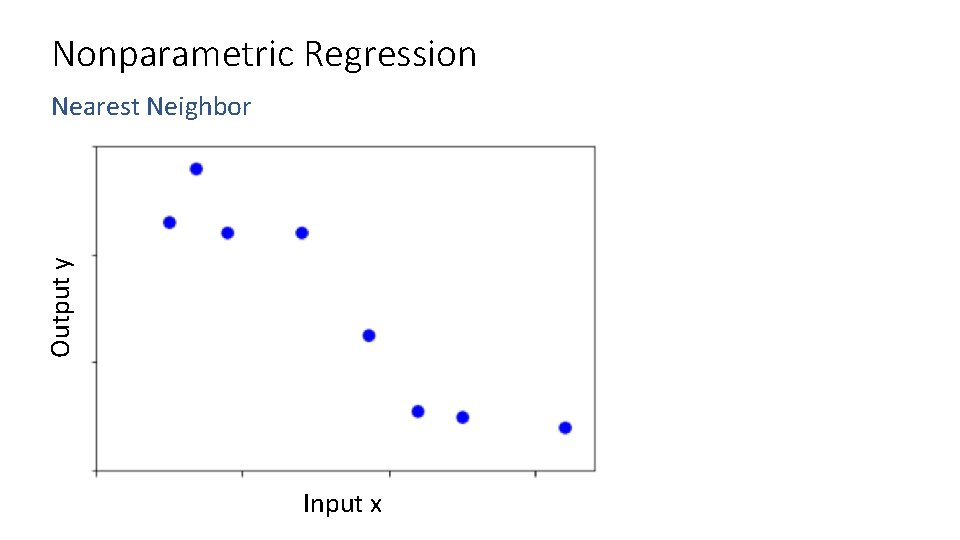Nonparametric Regression Output y Nearest Neighbor Input x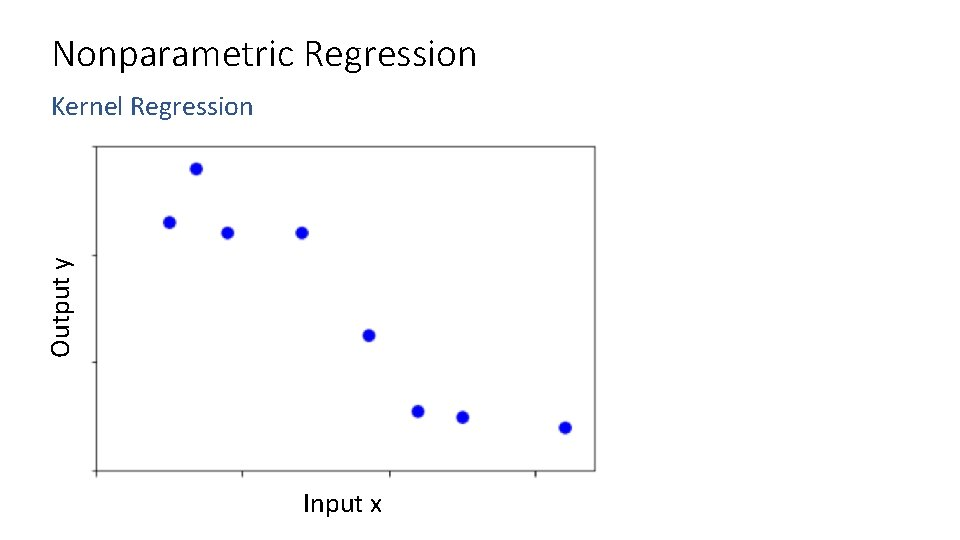Nonparametric Regression Output y Kernel Regression Input xNonparametric Regression Output y Kernel Regression Input xKernel RegressionKernel Regression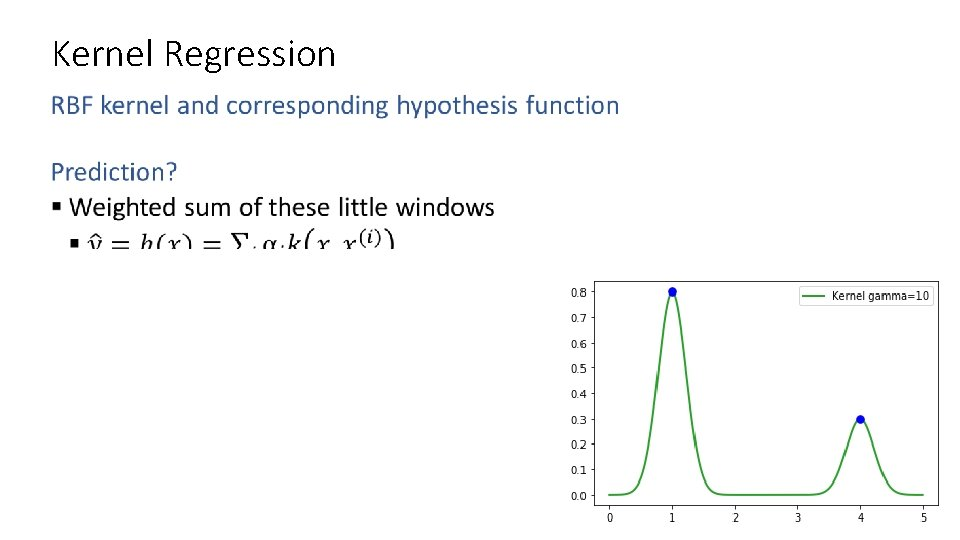Kernel Regression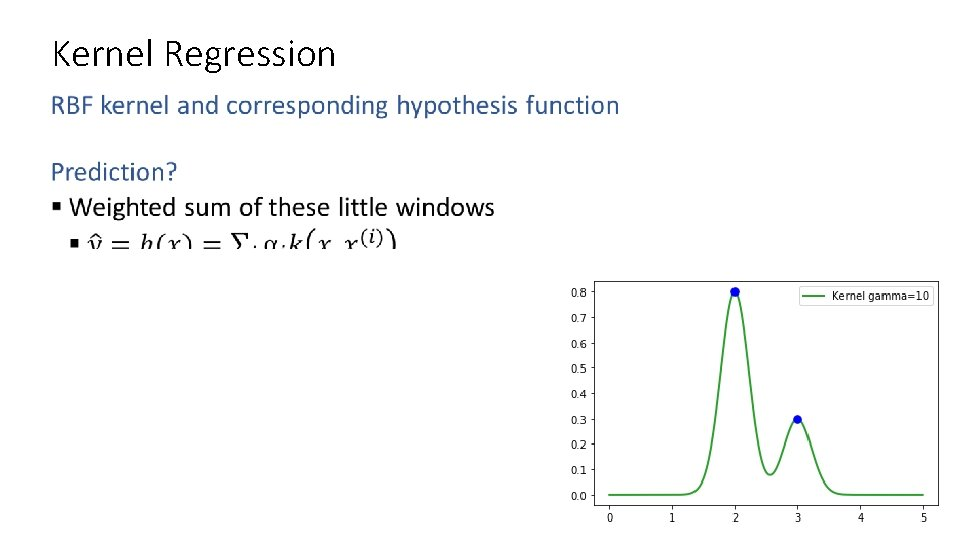Kernel Regression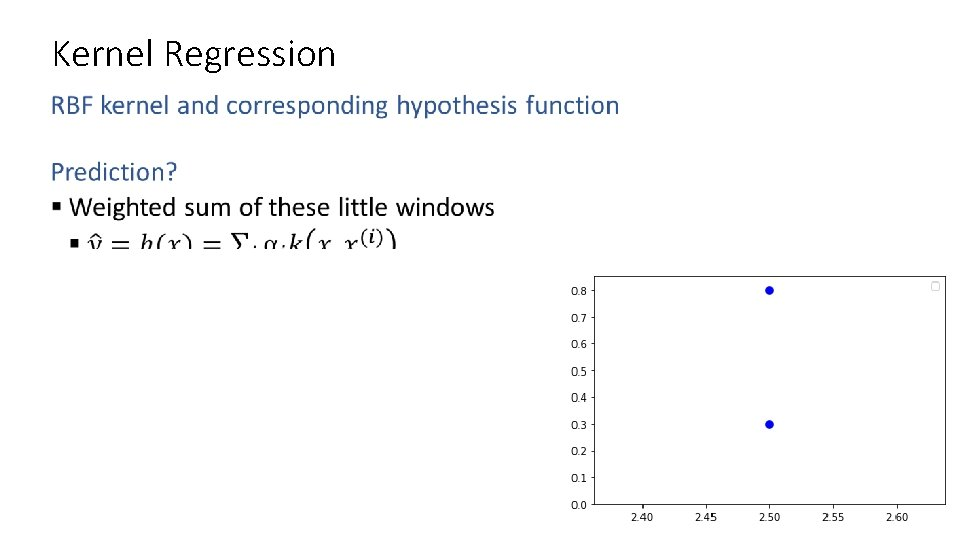Kernel Regression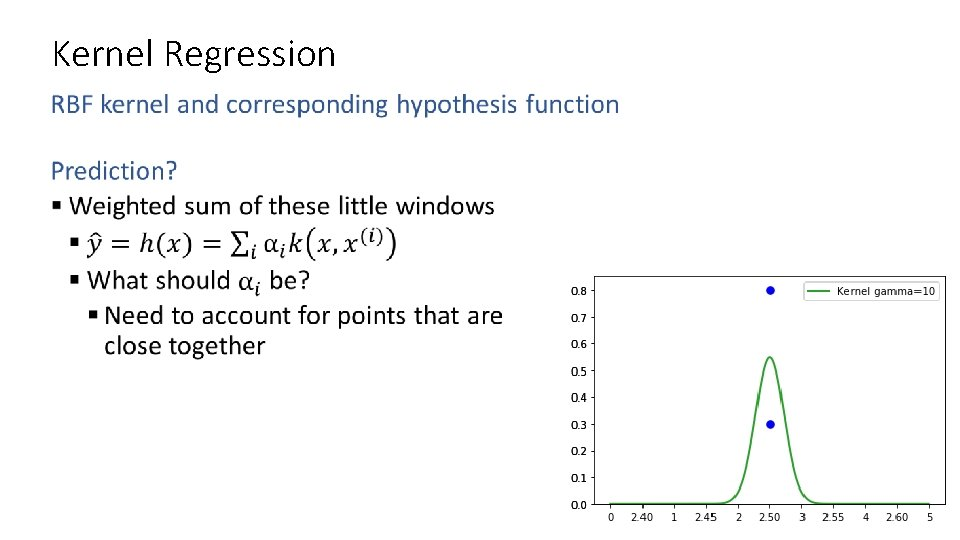Kernel RegressionKernel Regression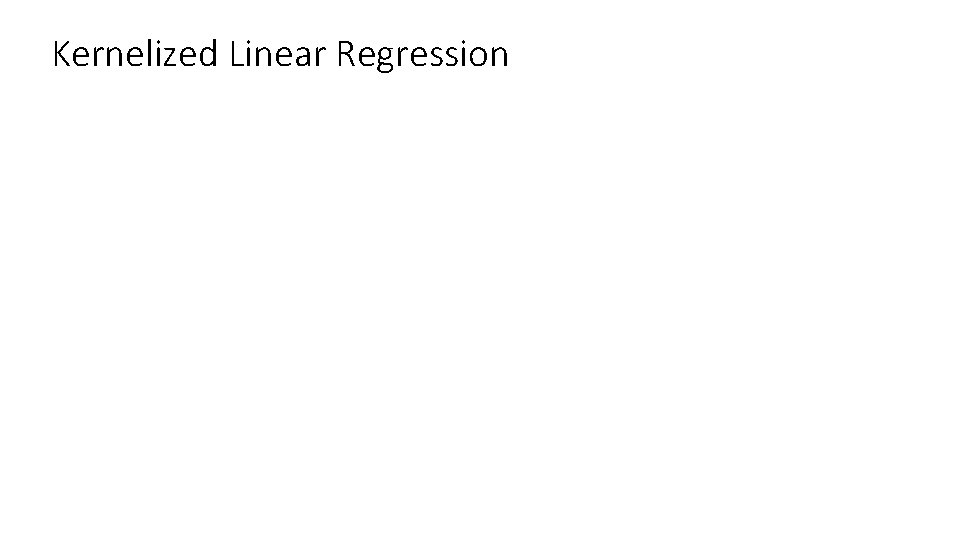Kernelized Linear Regression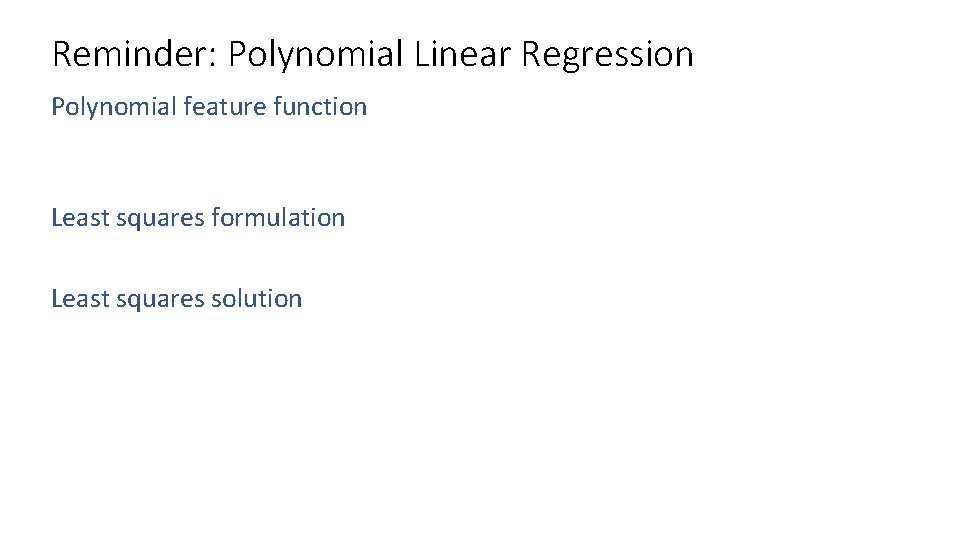Reminder: Polynomial Linear Regression Polynomial feature function Least squares formulation Least squares solutionReminder: Polynomial Linear RegressionKernelized Linear Regression